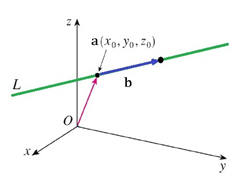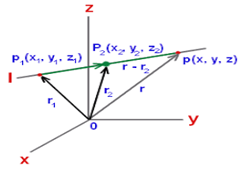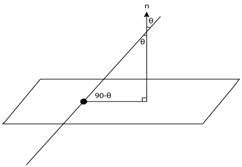Q

# Can someone explain - The equationof the line passing throughparallel to the planeand intersecting the lineis : - Three Dimensional Geometry - JEE Main

The equation of the line passing through  parallel to the plane  and intersecting the line  is :

• Option 1)

• Option 2)

• Option 3)• Option 4)Views

Cartesian eqution of a line -

The equation of a line passing through two points and parallel to vector having direction ratios as is given by

The equation of a line passing through two points  is given by

- whereinAngle between line and Plane (vector form ) -

The angle between a line and the plane is given by

- whereinFrom the concept we have learnt

Normal vector of plane containing two intersecting  lines is parallel to vector

Required line is parallel to vector

Required equation of line is

Option 1)

Option 2)

Option 3)Option 4)Exams
Articles
Questions